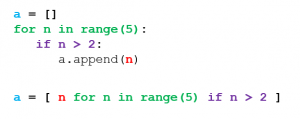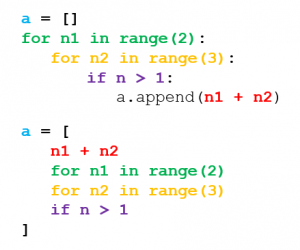# List Comprehension in Python Explained Visually

In Python, a list is denoted with two brackets `[]`.

There are three ways you can populate a list:

## Method 1: Initialize a List Literal

A literal simply refers to an expression that can be used as-is. No variables. No fancy lingo.

In a list literal, all the items in the list are explicitly declared upon initialization.

``````a = [0, 1, 2, 3, 4]
``````

This is the simplest way to populate a list.

## Method 2: Fill an Empty List

The second way to add items to a list first involves creating an empty list using the double brackets. In order to populate the list, we can write a for loop that will add numbers to the list.

``````a = [] # empty list
for num in range(5): # [0,5)
a.append(num)
``````

## Method 3: Use List Comprehension

The final way to populate a list is to write the for loop expression _inside_the brackets upon initialization.

``````a = [num for num in range(5)]
``````

## How Does This Work?

List comprehension is a concise way of creating a list. It’s a Python-specific feature that does not add any functionality to the language but rather improves code readability.

### Conversion 1: Unconditional for-loop

The best way to think about list comprehension is to think of it as a conversion from a for-loop that adds to a list.

``````a = []
for n in range(5): # [0,5)
a.append(n)
``````
``````a = [n for n in range(5)] # [0, 1, 2, 3, 4]
``````

As you can see, those three lines on the left can be condensed to the single line on the right.

There is no need to declare an empty list.

There is no need to `append()` to the list. That functionality is inherent in list comprehension.

### Conversion 2: Conditional for-loop

Suppose you have a condition (if-statement) that you want to include in the for-loop. That can be added at the end of the expression inside the brackets.

``````a = []
for n in range(5): # [0,5)
if n > 2:
a.append(n)
``````
``````a = [n for n in range(5) if n > 2] # [3, 4]
``````

### Conversion 3: Nested for-loop

If we wanted to run another for-loop inside the original for-loop, we can simply add that expression at the end as well.

``````a = []
for n1 in range(2): # [0,2)
for n2 in range(3): # [0,3)
a.append(n1 + n2)
``````
``````a = [n1 + n2 for n1 in range(2) for n2 in range(3)]
# [0, 1, 2, 1, 2, 3] = [0+0, 0+1, 0+2, 1+0, 1+1, 1+2]
``````

## Visualization

The following is a color visualization of the conversion to list comprehension.Remember that Python allows you to have multi-line expressions inside brackets, so you can utilize this feature if it improves readability. This is especially helpful for comprehensions with nested loops.## Conclusion

At the end of the day, list comprehensions allow for quicker development and better readability.